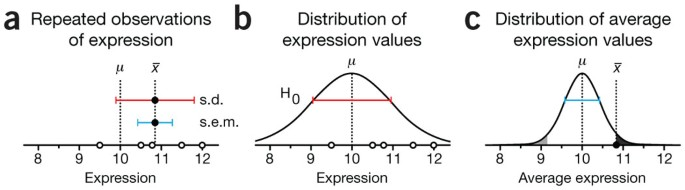Bench scientists often perform statistical tests to determine whether an observation is statistically significant. Many tests report the P value to measure the strength of the evidence that a result is not just a likely chance occurrence. To make informed judgments about the observations in a biological context, we must understand what the P value is telling us and how to interpret it. This month we will develop the concept of statistical significance and tests by introducing the one-sample t-test.

To help you understand how statistical testing works, consider the experimental scenario depicted in Figure 1 of measuring protein expression level in a cell line with a western blot. Suppose we measure an expression value of x = 12 and have good reason to believe (for example, from past measurements) that the reference level is μ = 10 (Fig. 1a). What can we say about whether this difference is due to random chance? Statistical testing can answer this question. But first, we need to mathematically frame our intuitive understanding of the biological and technical factors that disperse our measurements across a range of values.

We begin with the assumption that the random fluctuations in the experiment can be characterized by a distribution (Fig. 1b). This distribution is called the null distribution, and it embodies the null hypothesis (H0) that our observation is a sample from the pool of all possible instances of measuring the reference. We can think of constructing this distribution by making a large number of independent measurements of a protein whose mean expression is known to equal the reference value. This distribution represents the probability of observing a given expression level for a protein that is being expressed at the reference level. The mean of this distribution, μ, is the reference expression, and its spread is determined by reproducibility factors inherent to our experiment. The purpose of a statistical test is to locate our observation on this distribution to identify the extent to which it is an outlier.

Statistics quantifies the outlier status of an observation by the probability of sampling another observation from the null distribution that is as far or farther away from μ. In our example, this corresponds to measuring an expression value further from the reference than x. This probability is the P value, which is the output of common statistical tests. It is calculated from the area under the distribution curve in the shaded regions (Fig. 1c). In some situations we may care only if x is too big (or too small), in which case we would compute the area of only the dark (light) shaded region of Figure 1c.

Unfortunately, the P value is often misinterpreted as the probability that the null hypothesis (H0) is true. This mistake is called the 'prosecutor's fallacy', which appeals to our intuition and was so coined because of its frequent use in courtroom arguments. In the process of calculating the P value, we assumed that H0 was true and that x was drawn from H0. Thus, a small P value (for example, P = 0.05) merely tells us that an improbable event has occurred in the context of this assumption. The degree of improbability is evidence against H0 and supports the alternative hypothesis that the sample actually comes from a population whose mean is different than μ. Statistical significance suggests but does not imply biological significance.

At this point you may ask how we arrive at our assumptions about the null distribution in Figure 1b. After all, in order to calculate P, we need to know its precise shape. Because experimentally determining it is not practical, we need to make an informed guess. For the purposes of this column, we will assume that it is normal. We will discuss robustness of tests to this assumption of normality in another column. To complete our model of H0, we still need to estimate its spread. To do this we return to the concept of sampling.

To estimate the spread of H0, we repeat the measurement of our protein's expression. For example, we might make four additional independent measurements to make up a sample with n = 5 (Fig. 2a). We use the mean of expression values ($$\overline{x}$$ = 10.85) as a measure of our protein's expression. Next, we make the key assumption that the s.d. of our sample (sx = 0.96) is a suitable estimate of the s.d. of the null distribution (Fig. 2b). In other words, regardless of whether the sample mean is representative of the null distribution, we assume that its spread is. This assumption of equal variances is common, and we will be returning to it in future columns.Figure 2: Repeated independent observations are used to estimate the s.d. of the null distribution and derive a more robust P value.

From our discussion about sampling1, we know that given that H0 is normal, the sampling distribution of means will also be normal, and we can use sx/√n to estimate its s.d. (Fig. 2c). We localize the mean expression on this distribution to calculate the P value, analogously to what was done with the single value in Figure 1c. To avoid the nuisance of dealing with a sampling distribution of means for each combination of population parameters, we can transform the mean to a value determined by the difference of the sample and population means D = μ divided by the s.e.m. (sx/√n). This is called the test statistic.

It turns out, however, that the shape of this sampling distribution is close to, but not exactly, normal. The extent to which it departs from normal is known and given by the Student's t distribution (Fig. 3a), first described by William Gosset, who published under the pseudonym 'Student' (to avoid difficulties with his employer, Guinness) in his work on optimizing barley yields. The test statistic described above is compared to this distribution and is thus called the t statistic. The test illustrated in Figure 2 is called the one-sample t-test.